Start typing, then use the up and down arrows to select an option from the list.# Reaction Mechanism Example 2

Jules Bruno
231views
the elementary reaction of two moles of nitrogen dioxide reacting with one mole of flooring gas to produce two moles of N. 02 F gas is an example of a blank type of reaction. All right, so here we're talking about molecular charity. So, we need to determine the total number of reactant moles involved. So here we have two moles of nitrogen dioxide and one mole of flooring gas Together that totals three moles of reactant. So unique molecular means we only have one mole of of reactant by molecular would mean to Tetra would mean four. So, the answer is either B or deep. So it's either try molecular or term molecular. Remember from our earlier videos when it comes to molecular charity, three moles of reactant is referred to as term molecular. Try molecular does not exist. So here the answer would be option deep.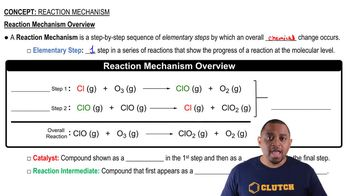03:06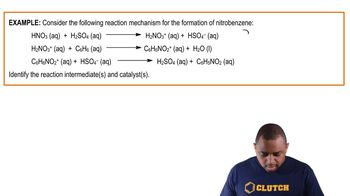02:14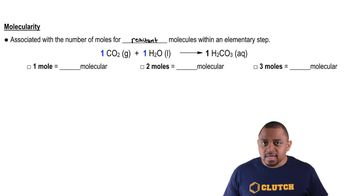01:31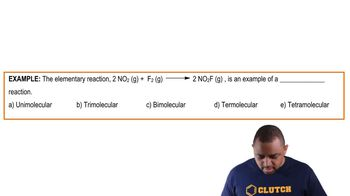01:02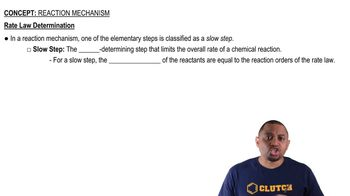00:58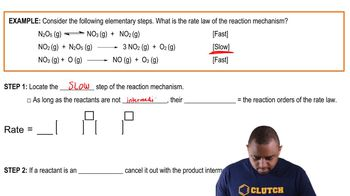04:16